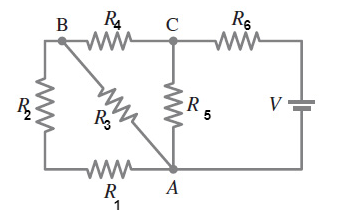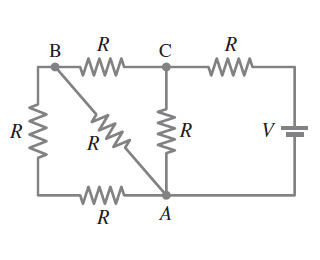# Problem: What is the net resistance of the circuit connected to the battery in (Figure 1)?Express your answer in terms of R.

###### FREE Expert Solution

Equivalent resistance for resistors in series:

$\overline{){{\mathbf{R}}}_{\mathbf{e}\mathbf{q}}{\mathbf{=}}{{\mathbf{R}}}_{{\mathbf{1}}}{\mathbf{+}}{{\mathbf{R}}}_{{\mathbf{2}}}{\mathbf{+}}{\mathbf{.}}{\mathbf{.}}{\mathbf{.}}{\mathbf{+}}{{\mathbf{R}}}_{{\mathbf{n}}}}$

Equivalent resistor for resistors in parallel:

$\overline{)\frac{\mathbf{1}}{{\mathbf{R}}_{\mathbf{e}\mathbf{q}}}{\mathbf{=}}\frac{\mathbf{1}}{{\mathbf{R}}_{\mathbf{1}}}{\mathbf{+}}\frac{\mathbf{1}}{{\mathbf{R}}_{\mathbf{2}}}{\mathbf{+}}{\mathbf{.}}{\mathbf{.}}{\mathbf{.}}{\mathbf{+}}\frac{\mathbf{1}}{{\mathbf{R}}_{\mathbf{n}}}}$

Let's label the resistors as follows:Resistors 1 and 2 are in series.

R12 = R1 + R2 = R + R = 2R

R12 and R3 are in parallel.

1/R123 = 1/2R + 1/R = 3/2R###### Problem Details

What is the net resistance of the circuit connected to the battery in (Figure 1)?